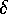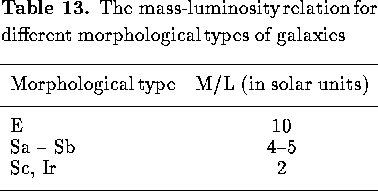Next: Gravitational wave background from Previous: Gravitational waves from supernova

## Gravitational Waves from Coalescing Compact Binary Stars

The GW from coalescing compact binaries composed of NS and BH are the best understood of all astrophysical GW sources. A conservative lower limit to the event rate of galactic binary neutron star coalescence of about 1 per100000 year follows from double pulsarĀ statistics studies (Narayan et al., 1991; Phinney, 1991). Theoretical estimates, however, give much higher values, of about 1 per3000 - 10000 year (Lipunov et al., 1987a; Tutukov and Yungelson, 1993a; Lipunov et al., 1995e). Observational estimates are subject to (unknown) selection effects, whereas theoretical estimates based on evolutionary studies of binary stars use a number of poorly known parameters of binary evolution, such as the initial binary mass distribution exponent(see SectionĀ7).

In FigureĀ47 we show the rates of coalescence of binary WD and NS in a model elliptical galaxyĀ consisting ofstars, depending on time elapsed since the (assumed instantaneous) star formation. Calculations were made with the adopted best values of key evolutionary parameters (Lipunov et al., 1995d; and SectionĀ7). We note that the coalescence rate of double WD1 per 100 year only slightly depends on the parameters, making these events an attractive mechanism for supernovae of type 1a observed in elliptical galaxiesĀ at nearly the same rates (Iben and Tutukov 1984a,b[72, 73]). Evolution of supernova rates in elliptical galaxiesĀ was first modeled by Lipunov and Postnov (1988). Unlike double WD, the coalescenceĀ rate of double NS evolves significantly with time.

ĀFigure 47: Evolution of coalescence rates of WD+WD and NS+NS binaries with time in a model elliptical galaxy with a mass of(Lipunov et al., 1995a).Ā

Having calculated the evolution of binaries in the model elliptical galaxyĀ with a-function-like star formation, one can easily model evolution of a galaxy with an arbitrary time dependence of star formation rate. For example, a normal spiral galaxyĀ can be approximated as having a constant star formation rate. In this case our calculations give a coalescence rate of double NS of 1 per4000 Āyear.

The next obvious step was to calculate the distribution of the binary NS coalescence event rates on the sky, using Tully's Nearby Galaxies Catalog. Since nothing is known about the initial binary distributions in other galaxies, we have assumed that the initial mass functionof binary components and initial semi-major axes distribution f(a) of binaries in all galaxies are similar to those observed in our Galaxy:Ā

The total number of binaries in a galaxy must be proportional to its total mass, which can be estimated by using an average mass-luminosity relation for galaxies of different morphological types. We adopted the M/L relation following Sil'chenko (1984) (see TableĀ14):

ĀThese values give estimations of the mass of the stellar component of galaxies (dark matter halo is not included) to an accuracy of factor 2. Star formation rates were taken to be constant for both spiralĀ and irregularĀ galaxies; for elliptical galaxiesĀ the star formation rate was assumed to be constant and nonzero only during the first billion years.

The resulting map of coalescence rate of binary NS is presented in FigureĀ48 in terms of events per one square degree per year. The event rate integrated over the whole sky is3 per year. This is a very optimistic estimate for the LIGO experiments.

ĀFigure 48: Coalescence rate of binary NS in terms of events per one square degree per year in galactic coordinates. Integral event rate is3 events per year (Lipunov et al., 1995a).ĀNext: Gravitational wave background from Previous: Gravitational waves from supernova

Mike E. Prokhorov
Sat Feb 22 18:38:13 MSK 1997